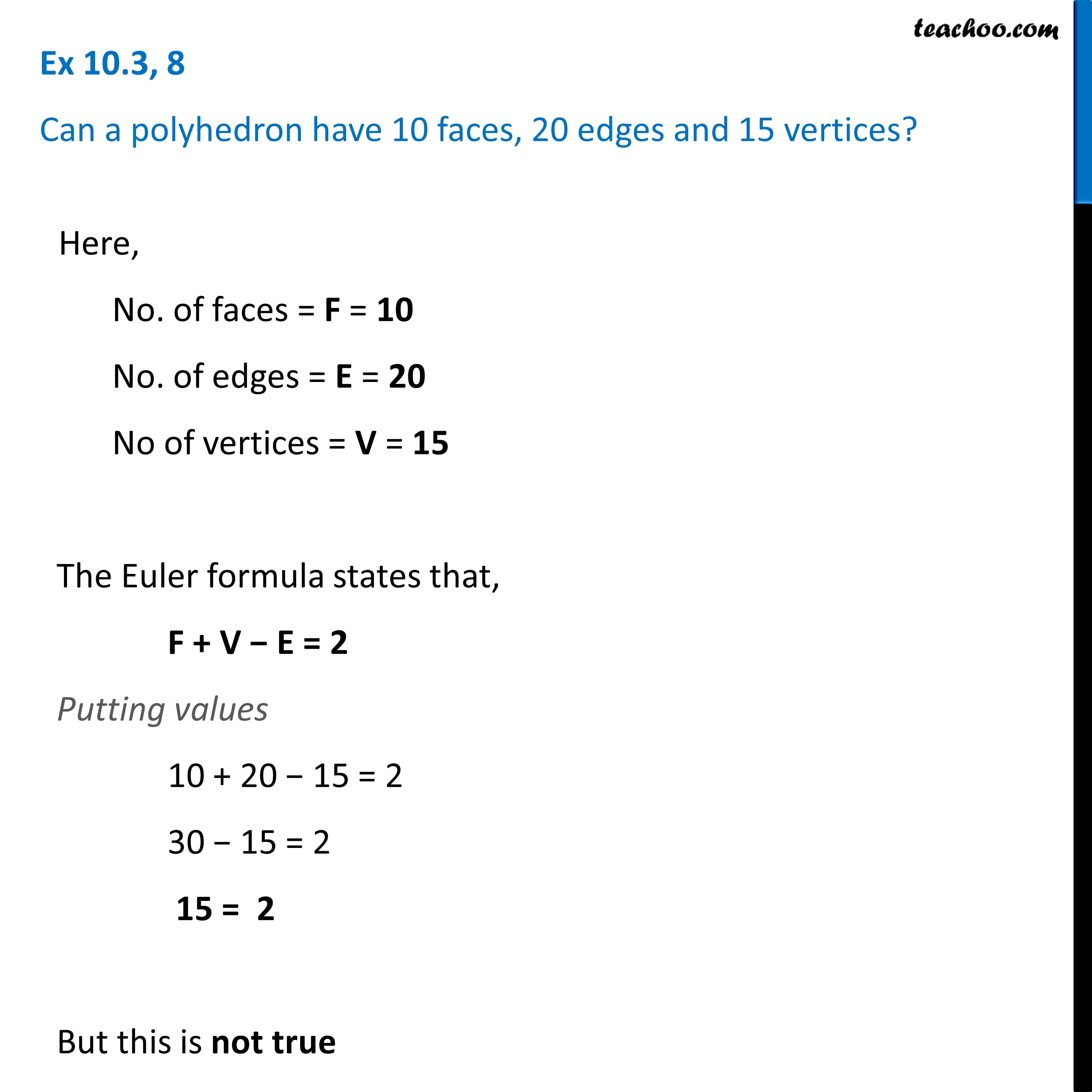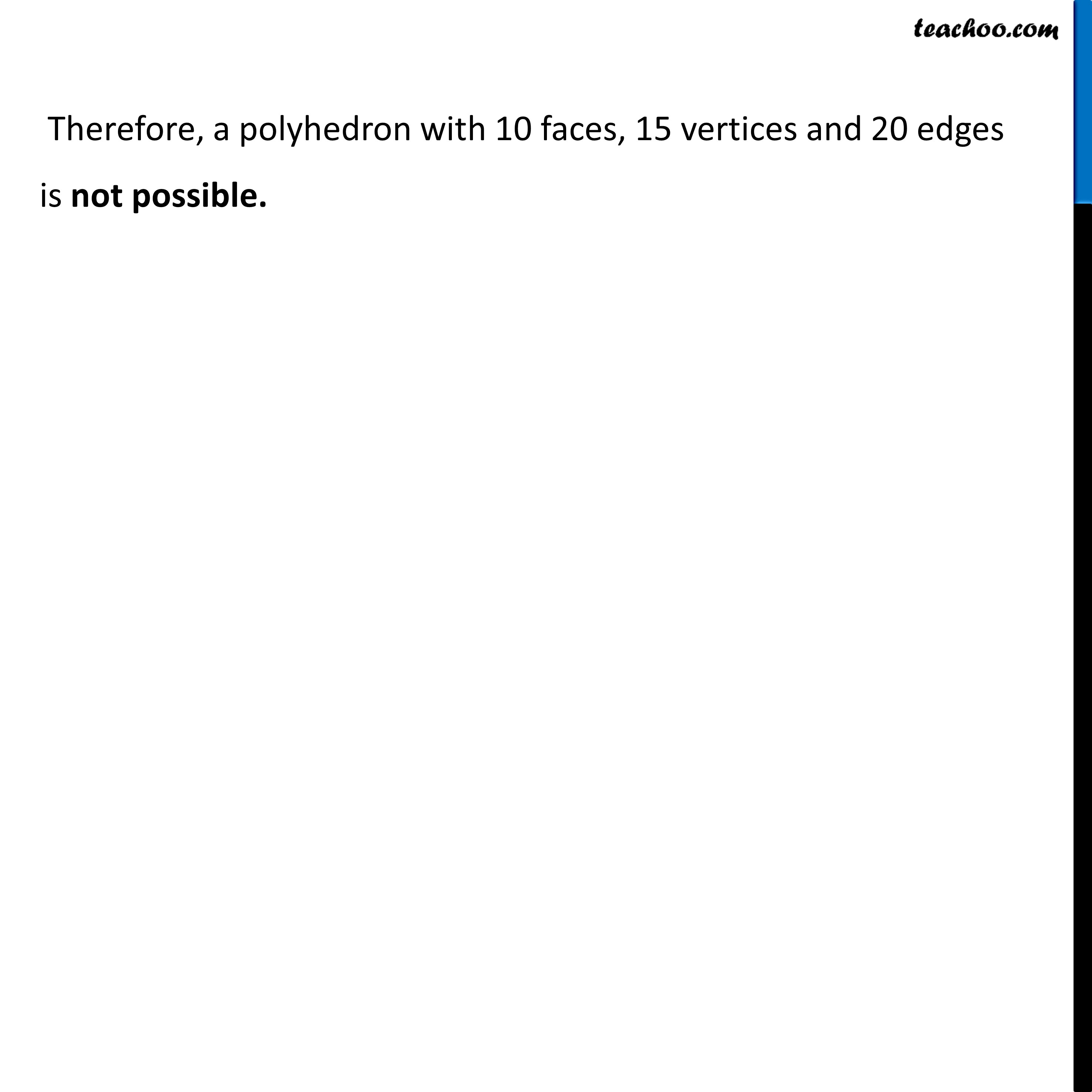Prism and Polyhedrons

Visualising Solid Shapes
Serial order wiseLearn in your speed, with individual attention - Teachoo Maths 1-on-1 Class

### Transcript

Question 8 Can a polyhedron have 10 faces, 20 edges and 15 vertices?Here, No. of faces = F = 10 No. of edges = E = 20 No of vertices = V = 15 The Euler formula states that, F + V − E = 2 Putting values 10 + 20 − 15 = 2 30 − 15 = 2 15 = 2 But this is not true